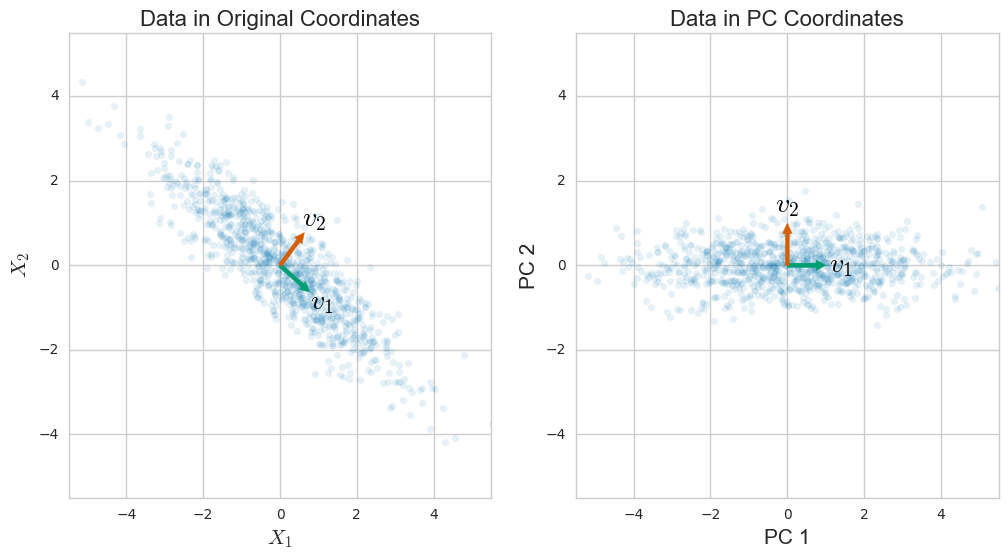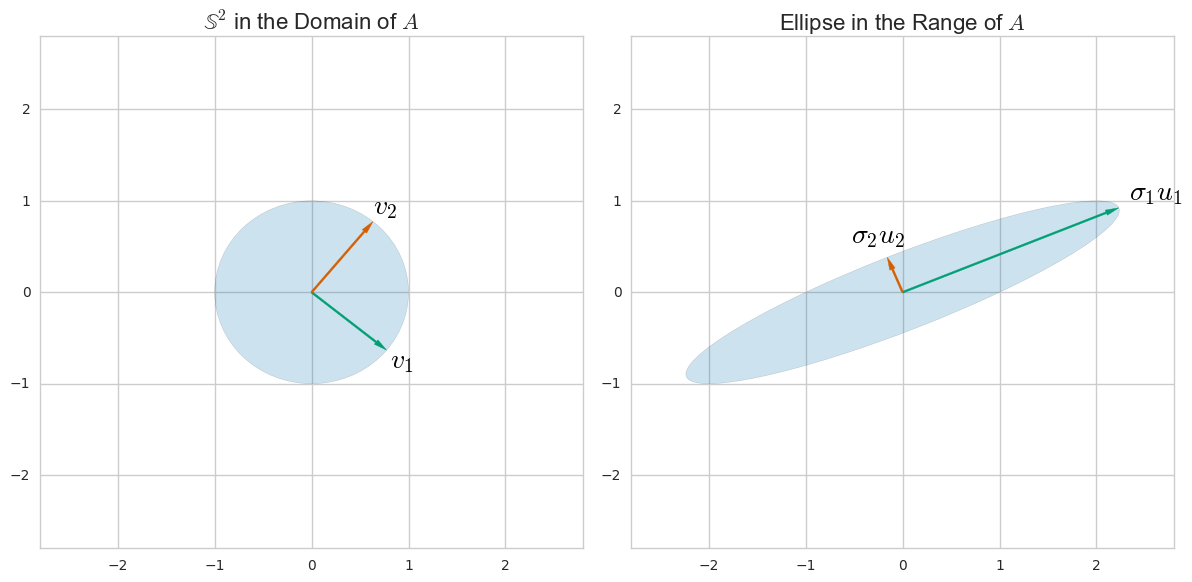# Intuitive explanation of difference between PCA and SVD [closed]

Can someone explain the difference between SVD and PCA with real life example?

• Jan 4, 2020 at 18:26

PCA. Suppose that you have n data points comprised of d numbers (or dimensions) each. If you center this data (subtract the mean data point $$\mu$$ from each data vector $$x_i$$) you can stack the data to make a matrix

$$X = \left( \begin{array}{ccccc} && x_1^T - \mu^T && \\ \hline && x_2^T - \mu^T && \\ \hline && \vdots && \\ \hline && x_n^T - \mu^T && \end{array} \right)\,.$$

The covariance matrix

$$S = \frac{1}{n-1} \sum_{i=1}^n (x_i-\mu)(x_i-\mu)^T = \frac{1}{n-1} X^T X$$

measures to which degree the different coordinates in which your data is given vary together. So, it's maybe not surprising that PCA -- which is designed to capture the variation of your data -- can be given in terms of the covariance matrix. In particular, the eigenvalue decomposition of $$S$$ turns out to be

$$S = V \Lambda V^T = \sum_{i = 1}^r \lambda_i v_i v_i^T \,,$$

where $$v_i$$ is the $$i$$-th Principal Component, or PC, and $$\lambda_i$$ is the $$i$$-th eigenvalue of $$S$$ and is also equal to the variance of the data along the $$i$$-th PC. This decomposition comes from a general theorem in linear algebra, and some work does have to be done to motivate the relatino to PCA.SVD is a general way to understand a matrix in terms of its column-space and row-space. (It's a way to rewrite any matrix in terms of other matrices with an intuitive relation to the row and column space.) For example, for the matrix $$A = \left( \begin{array}{cc}1&2\\0&1\end{array} \right)$$ we can find directions $$u_i$$ and $$v_i$$ in the domain and range so thatYou can find these by considering how $$A$$ as a linear transformation morphs a unit sphere $$\mathbb S$$ in its domain to an ellipse: the principal semi-axes of the ellipse align with the $$u_i$$ and the $$v_i$$ are their preimages.

In any case, for the data matrix $$X$$ above (really, just set $$A = X$$), SVD lets us write

$$X = \sum_{i=1}^r \sigma_i u_i v_j^T\,,$$

where $$\{ u_i \}$$ and $$\{ v_i \}$$ are orthonormal sets of vectors.A comparison with the eigenvalue decomposition of $$S$$ reveals that the "right singular vectors" $$v_i$$ are equal to the PCs, the "right singular vectors" are

$$u_i = \frac{1}{\sqrt{(n-1)\lambda_i}} Xv_i\,,$$

and the "singular values" $$\sigma_i$$ are related to the data matrix via

$$\sigma_i^2 = (n-1) \lambda_i\,.$$

It's a general fact that the right singular vectors $$u_i$$ span the column space of $$X$$. In this specific case, $$u_i$$ give us a scaled projection of the data $$X$$ onto the direction of the $$i$$-th principal component. The left singular vectors $$v_i$$ in general span the row space of $$X$$, which gives us a set of orthonormal vectors that spans the data much like PCs.

I go into some more details and benefits of the relationship between PCA and SVD in this longer article.

Original post on crossvalid